The Sine Law in terms of Circum-Radius R.﻿
Statement: In any ΔABC,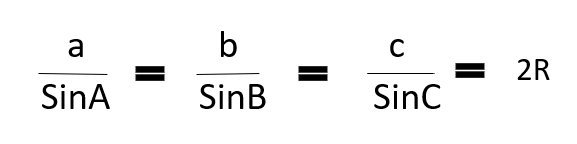where R is the Circum-Radius of ΔABC.

Proof:
Consider the circum-circle of ΔABC with center O and radius R. Then we can have three possible figures.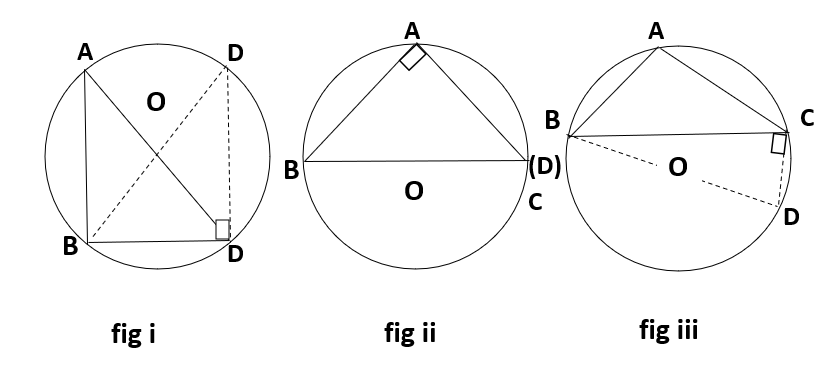Where the angle A is acute in fig i, or right angled in fig ii or obtuse in fig iii.
Let O be the center of the circum-circle of ΔABC and R be the circum-radius. Join BO and produce it to meet the circle at D.

Now in figure i,
BAC = BDC = A, BCD = 90º (angle at the semicircle).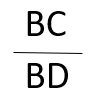= Sin(BDC)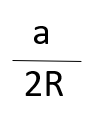= SinA
↣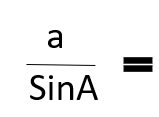2R

In fig iii,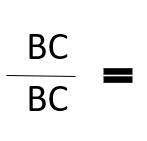1 = Sin90º = SinA
↣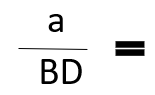SinA ( Since BC = BD )
↣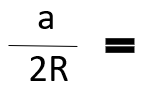SinA
↣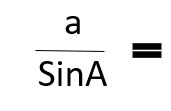2R

In fig iii,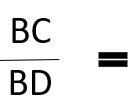SinA
↣SinA2R

Therefore in each figure it is found that2R
Similarly we can prove that: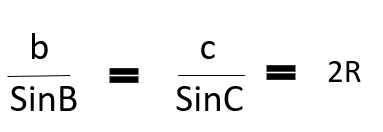Combining these we get,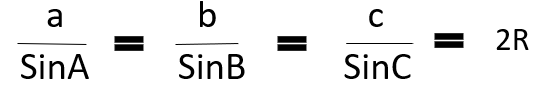Proved.